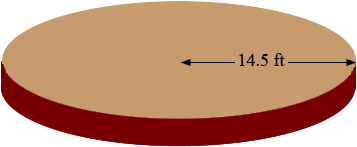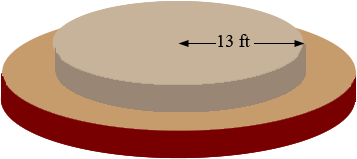SEARCH HOMEMath Central Quandaries & QueriesQuestion from PATTY: POOL RADIUS IS 13 FEET ON THE OUTER EDGE, I WANT TO MAKE AN 18 INCH OUTSIDE BORDER AROUND THE POOL. WHAT IS THE VOLUME AROUND THE POOL. (THIS IS GOING FROM A 13 FT. RADIUS TO A 14.5 RADIUS.Patty,

You didn't tell us the thickness of the border, but if you know the thickness I can help you calculate the volume.

First use our volume calculator to calculate the volume of a cylinder of radius 14.5 feet and the thickness you want.Use our volume calculator again to calculate the volume of a cylinder with the same thickness and radius 13 feet.The volume of the border is the difference between the volumes of the large cylinder and the small cylinder.

I hope this helps,
PennyMath Central is supported by the University of Regina and The Pacific Institute for the Mathematical Sciences.